Custom SearchSIMILAR RIGHT TRIANGLES Two right triangles are SIMILAR if one of the acute angles of the first is equal to one of the acute angles of the second. This conclusion is supported by the following reasons: 1. The right angle in the first triangle is equal to the right angle in the second, since all right angles are equal. 2. The sum of the angles of any triangle is 180°. Therefore, the sum of the two acute angles in a right triangle is 90°. 3. Let the equal acute angles in the two triangles be represented by A and A’ respectively. (See fig. 19-4.) Then the other acute angles, B and B’, are as follows:         B = 90° - A         B’ = 90° - A’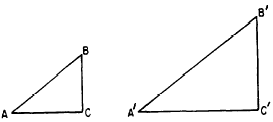Figure 19-4.-Similar right triangles. 4. Since angles A and A’ are equal, angles B and B’ are also equal. 5. We conclude that two right triangles with one acute angle of the first equal to one acute angle of the second have all of their corresponding angles equal. Thus the two triangles are similar. Practical situations frequently occur in which similar right triangles are used to solve problems. For example, the height of a tree can be determined by comparing the length of its shadow with that of a nearby flagpole, as shown in figure 19-5.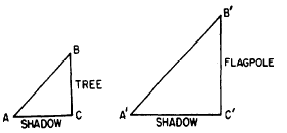Figure 19-5.-Calculation of height by comparison of shadows. Assume that the rays of the sun are parallel and that the tree and flagpole both form 90° angles with the ground. Then triangles ABC and A’B’C’ are right triangles and angle B is equal to angle B’ . Therefore, the triangles are similar and their corresponding sides are proportional, with the following result: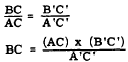Suppose that the flagpole is known to be 30 feet high, the shadow of the tree is 12 feet long, and the shadow of the flagpole is 24 feet long. Then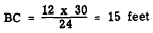Practice problems. 1. A mast at the top of a building casts a shadow whose tip is 48 feet from the base of the building. If the building is 12 feet high and its shadow is 32 feet long, what is the length of the mast? (NOTE: If the length of the mast is x, then the height of the mast above the ground is x + 12.) 2. Figure 19-6 represents an L-shaped building with dimensions as shown. On the line of sight from A to D, a stake is driven at C, a point 8 feet from the building and 10 feet from A. If ABC is a right angle, find the length of AB and the length of AD. Notice that AE is 18 feet and ED is 24 feet.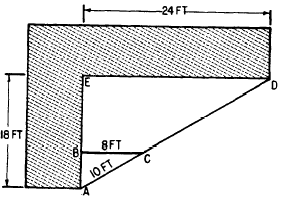Figure 19-6 - Using similar triangles. Answers: 1. 6 feet  2. AB = 6 feet     AD = 30 feet